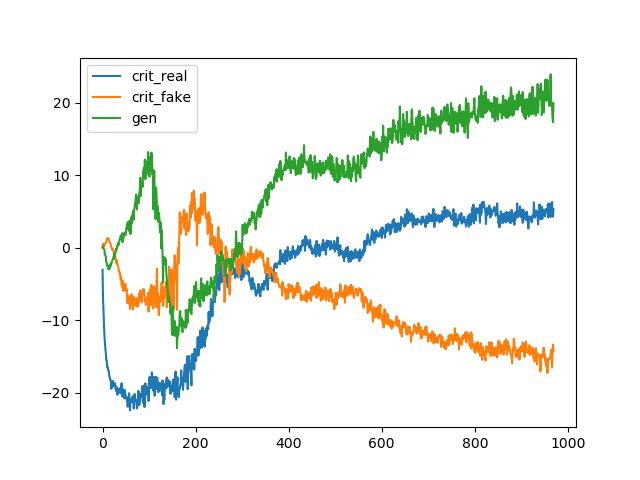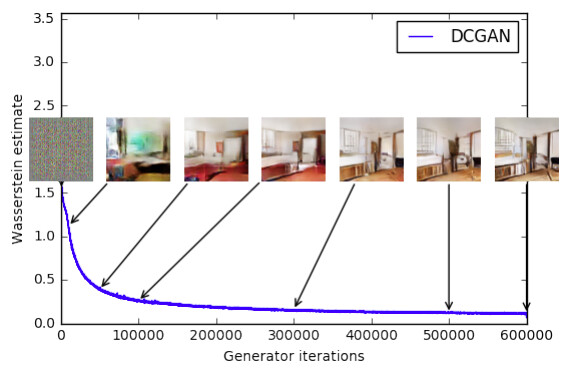# How can I calculate this steadily decreasing WGAN metric?

The loss of Wasserstein GANs is typically unconstrainted:(image source)

But I have seen examples of some WGAN metric that is steadily decreasing, for example thisfrom the original Wasserstein GAN paper. But how can this “Wasserstein metric” easily be calculated?

Generally, the discriminator loss is just D(x) - D(G(Z)) and the generator loss is D(G(Z)) but these produce losses akin to the top graph above. Admittedly I lack the math skills of understanding the original WGAN paper.